Pinewood Derby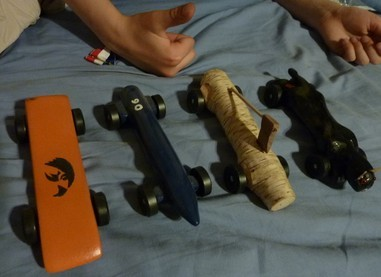Thumbs way up for the Pinewood Derby

The master equation

OK. If you are going for speed (uuh .. you are going for speed) …there is really one equation that describes everything.

where $v_{max}$ is the max speed of the car, $g$ is a constant, $H$ is the height of the car, $N$ is the number of wheels that are rotating, $m$ is the mass of one wheel and $M$ is the mass of car. In a game where every millisecond counts … punch this thing into a spreadsheet & start playing. Here is a screenshot of some realistic values showing the impact of several different design choices.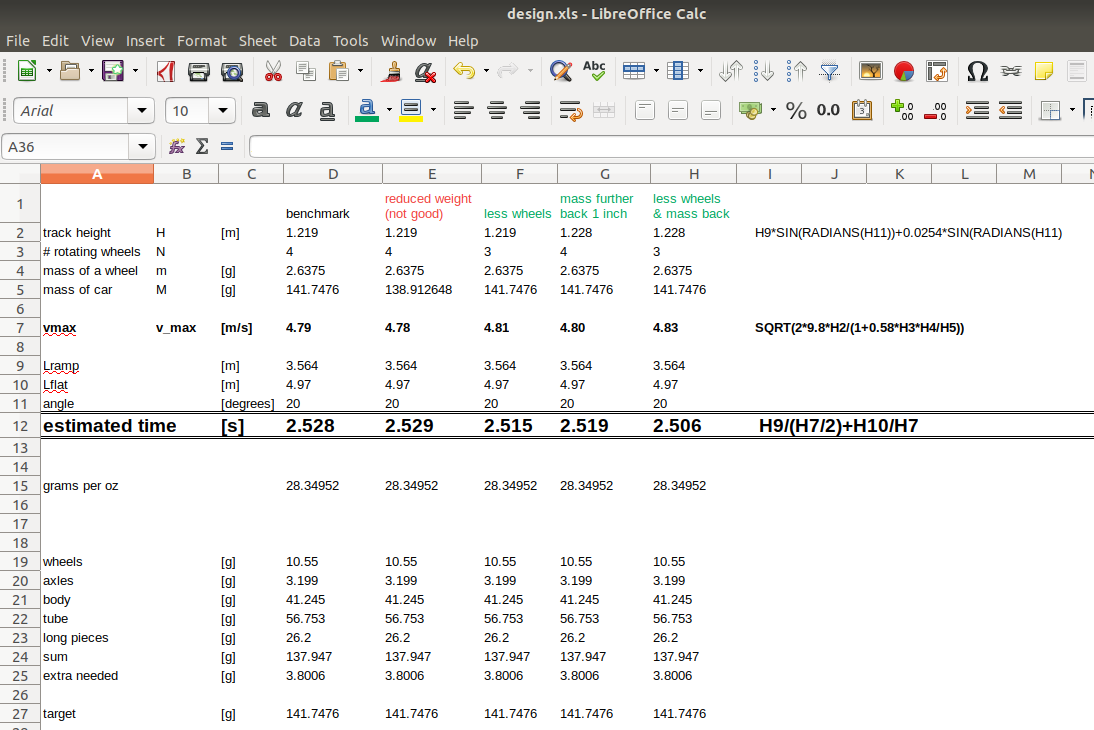Crunching the numbers

So what does it all mean?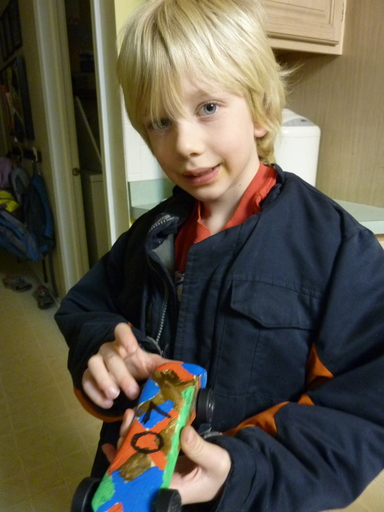Awesome … that’s what it all means

Besides having fun .. here are my 4 golden rules (plus one).

Do the basics right … de-burr the wheels, smooth the axles, graphite the axles & make sure the car rolls straight by testing and tweaking the axle positions. No extraneous friction.

The math says as large an $H$ as possible, but how? … Here’s how: get the extra weight you add as far back on the car as you can without it tipping over. This effectively “raises the height” of the car. Since the master equation is all about conversion of potential energy to kinetic energy, this is about the only (legal) way to do it.

The math says as large an $M$ as possible … so add weight right up to the max. This has nothing to do with heavier objects going faster (they don’t) .. it is because the larger the ratio $\frac{M}{m}$ the more of the original potential energy is converted into translational (vs. rotational) kinetic energy.

The math says as small an $N$ as possible. So $3$. So tweak it so only $3$ wheels touch the track … the real goal here is to keep one from not spinning & use that saved energy to propel the car forward (reduced friction is just a bonus)

+1: Make the car look awesome (for karma) … and also so the guy or gal staging them on the start ramp handles your torpedo with kid gloves.

Beyond these, everything else in the master equation ($g$, $m$) is fixed either by nature or the car kit you got at your cub scout troop.

If you can’t go fast …

Soooo … did we ever win the derby? Well, once or twice.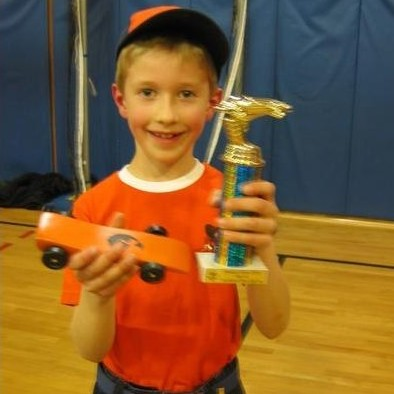Fast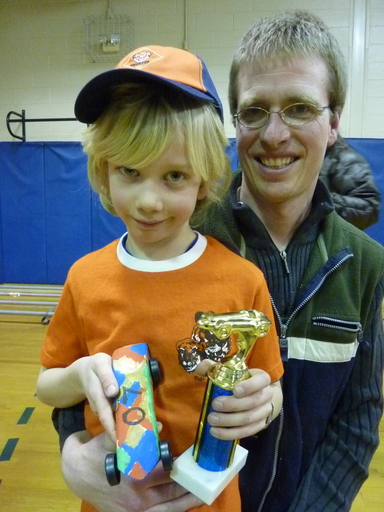Faster

We also got confused 1 a couple of years and got creative … and that worked out ok, too … turns out, there are points for style.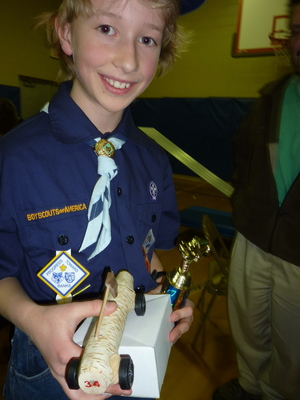The ol’ Adirondack log

Even more math

This gem goes into a ton more detail for all you budding Newtons out there.

1 see the opening sentence of the blog

Updated: